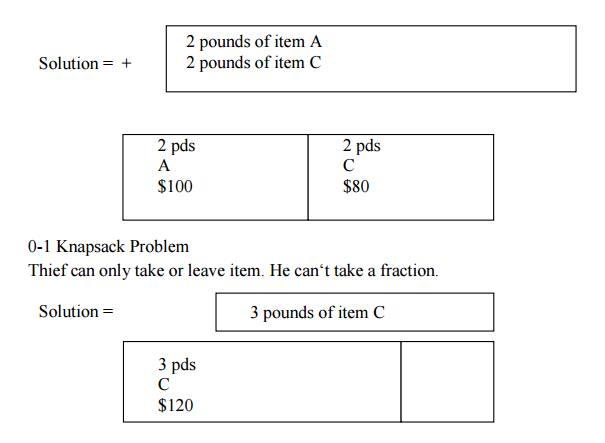Home | | User Interface Design | Hamiltonian Circuit Problem

# Hamiltonian Circuit Problem

As our next example, let us consider the problem of finding a Hamiltonian circuit in the graph of Figure 11.3a. Without loss of generality, we can assume that if a Hamiltonian circuit exists, it starts at vertex a.

HAMILTONIAN CIRCUIT PROBLEM

As our next example, let us consider the problem of finding a Hamiltonian circuit in the graph of Figure 11.3a. Without loss of generality, we can assume that if a Hamiltonian circuit exists, it starts at vertex a. Accordingly, we make vertex a the root of the state-space tree (Figure 11.3b).

The first component of our future solution, if it exists, is a first intermediate vertex of a Hamiltonian cycle to be constructed. Using the alphabet order to break the three-way tie among the vertices adjacent to a, we select vertex b. From b, the algorithm proceeds to c, then to d, then to e, and finally to f, which proves to be a dead end. So the algorithm backtracks from f to e, then to d. and then to c, which provides the first alternative for the algorithm to pursue.

Going from c to e eventually proves useless, and the algorithm has to backtrack from e to c and then to b. From there, it goes to the vertices f, e, c, and d, from which it can legitimately return to a, yielding the Hamiltonian circuit a, b, f, e, c, d, a. If we wanted to find another Hamiltonian circuit, we could continue this process by backtracking from the leaf of the solution found.Figure 11.3: (a) Graph. (b) State-space tree for finding a Hamiltonian circuit. The numbers above the nodes of the tree indicate the order in which the nodes are generated.

KNAPSACK PROBLEM

The knapsack problem or rucksack problem is a problem in combinatorial optimization: Given a set of items, each with a weight and a value, determine the number of each item to include in a collection so that the total weight is less than a given limit and the total value is as large as possible.

It derives its name from the problem faced by someone who is constrained by a fixed-size knapsack and must fill it with the most useful items.

The problem often arises in resource allocation with financial constraints. A similar problem also appears in combinatorics, complexity theory, cryptography and applied mathematics.

The decision problem form of the knapsack problem is the question "can a value of at least V be achieved without exceeding the weight W?"

E.g. A thief enters a store and sees the following items:His Knapsack holds 4 pounds.

What should he steal to maximize profit?

Fractional Knapsack Problem

Thief can take a fraction of an item.Fractional Knapsack has a greedy solution

Sort items by decreasing cost per poundStudy Material, Lecturing Notes, Assignment, Reference, Wiki description explanation, brief detail
Analysis and Design of Algorithm : Backtracking : Hamiltonian Circuit Problem |